# Prism Shape

Here we will learn about prisms, including what prisms are, types of prisms, nets of prisms and how to find the volume and surface area of prisms.

There are also volume and surface area of prisms worksheets based on Edexcel, AQA and OCR exam questions, along with further guidance on where to go next if you’re still stuck.

## What is a prism shape?

A prism shape is a polyhedron (a 3D shape made from polygons) with a constant cross section through one dimension. A prism shape has two congruent faces (identical ends).

For example, below are three different types of prisms:

The name of the prism is represented by the shape of its cross section.

### Faces, edges and vertices

A prism can be characterised using three features: faces, vertices and edges:

• Face: a closed, flat surface surrounded by edges and vertices.
• Edge: connects two vertices with a straight line
• Vertex (pl. vertices): a point where two or more edges meet.

We can label the vertices (corners) of a prism to help us identify certain edges or faces. Below is an example of a triangular prism where the letters A F represent the 6 vertices of the prism:

Using this labelling we can identify lengths such as the length AB :

We can also identify faces such as the face ABC :

The number of faces, edges and vertices can vary depending on the prism. Let’s look at some examples:

A prism is irregular if the cross section is not a regular polygon. For example, the L-shaped prism above is an irregular prism because the cross section is not a regular polygon.

### What is a prism?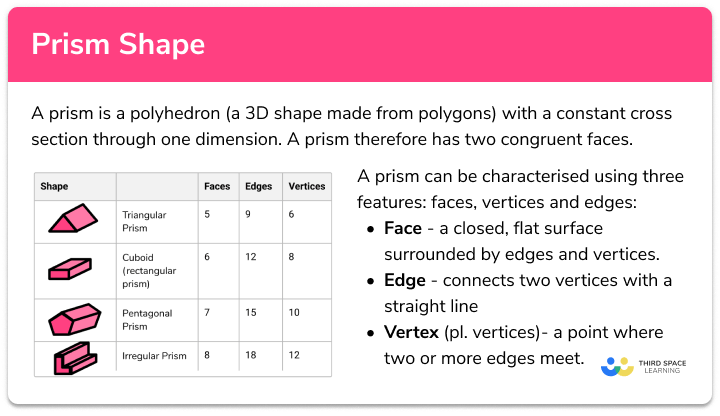### Nets

If you unfold a three-dimensional shape and lay it down so that it is flat, the result would be the net of the shape. We can draw a net for any prism.

E.g.

### Volume of a prism

The volume of a prism is how much space there is inside a prism.

To calculate the volume of a prism, we find the area of the cross section and multiply it by the depth.

Volume of prism = Area of cross section × Depth

Volume is measured in cubic units (e.g. mm^3, cm^3, m^3 etc).

Step-by-step guide: Volume of a prism

### Volume of a prism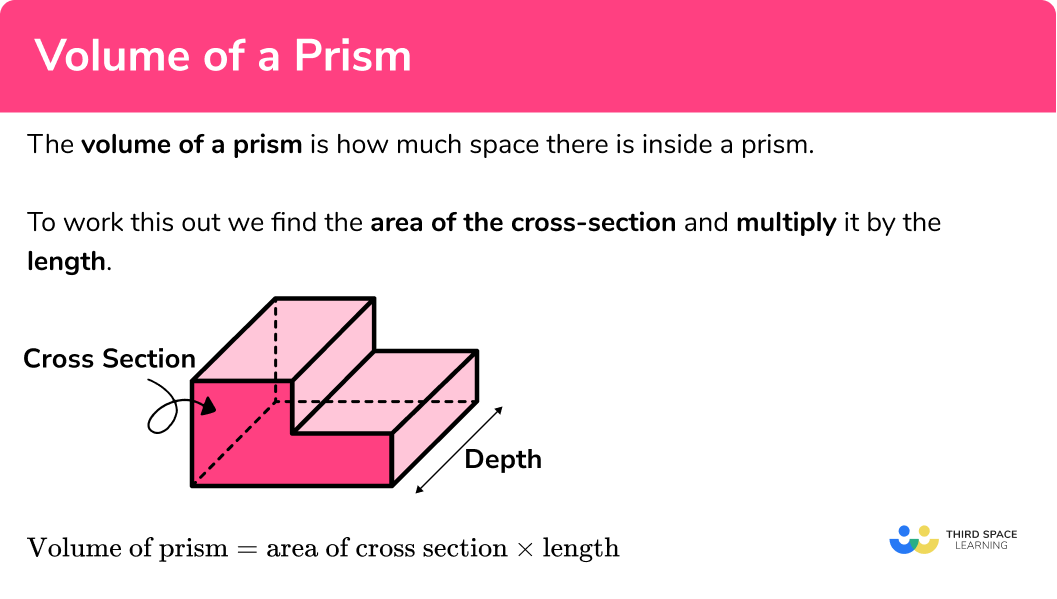## How to calculate the volume of a prism

In order to do this I need to follow the steps:

1. Write down the formula.
2. Calculate the area of the cross section.
3. Calculate the volume of the prism.
4. Write the answer, including the units.

### How to calculate the volume of a prism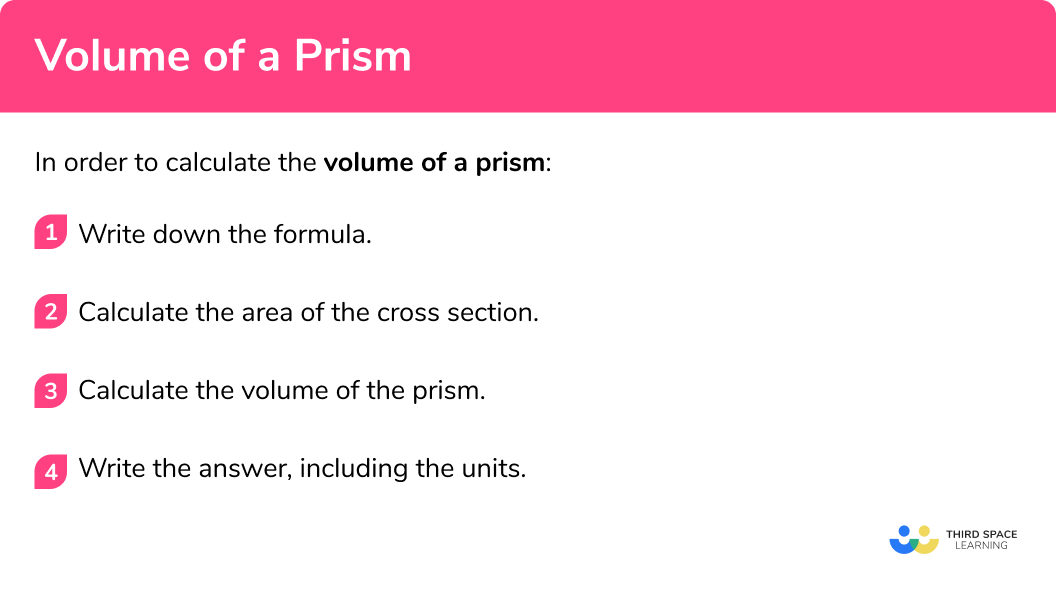## Volume of a prism examples

### Example 1: triangular prism, given the area of the cross-section

Below is a triangular prism:

The cross-sectional area of the prism is 43cm^2 . Calculate the volume of the prism.

1. Write down the formula.

Volume of prism = Area of cross section × Depth

2Calculate the area of the cross section.

The area of the cross section is already stated as 43cm^2 so we can move on to the next step.

3Calculate the volume of the prism.

$\text{Volume of prism }=\text{Area of cross section }\times\text{Depth}\\ =43\times{9}\\ =387$

4Write the answer, including the units.

The measurements on this prism are in cm so the volume will be measured in cm^3 .

Volume = 387cm^3 .

### Example 2: parallelogram prism

Work out the volume of the prism

1. Write down the formula.

Volume of prism = Area of cross section × Depth

2Calculate the area of the cross section.

$\text{Area of a parallelogram }=b\times{h}\\ =8\times{3}\\ =24$

3Calculate the volume of the prism.

$\text{Volume of prism }=\text{Area of cross section }\times{Depth}\\ =24\times{20}\\ =480$

4Write the answer, including the units.

The measurements on this prism are in m so the volume will be measured in m^3 .

Volume = 480m^3 .

### Example 3: trapezoidal prism

Calculate the volume of the prism below.

1. Write down the formula.

Volume of prism = Area of cross section × Depth

2Calculate the area of the cross section.

$\text{Area of cross section (trapezium) }=\frac{1}{2}(a+b)h\\ =\frac{1}{2}(5+9)\times{4}\\ =28\text{cm}^{2}$

3Calculate the volume of the prism.

$\text{Volume of prism }=\text{Area of cross section }\times\text{Depth}\\ =28\times{10}\\ =280$

4Write the answer, including the units.

Volume = 280cm^3

### Surface area of a prism

The surface area of a prism is the total area of all of the faces.

The surface area of a prism is special because all of the lateral faces are rectangles. To calculate the surface area of a prism, we calculate twice the area of the cross section and add the perimeter of the cross section multiplied by the depth of the prism.

In general, the surface area of any prism is:

Surface Area of a Prism = 2A+PD

where:

• A = Area of the cross section
• P = Perimeter of the cross section
• D = Depth of the prism

Step-by-step guide: Surface area of a prism

### Surface area of a prism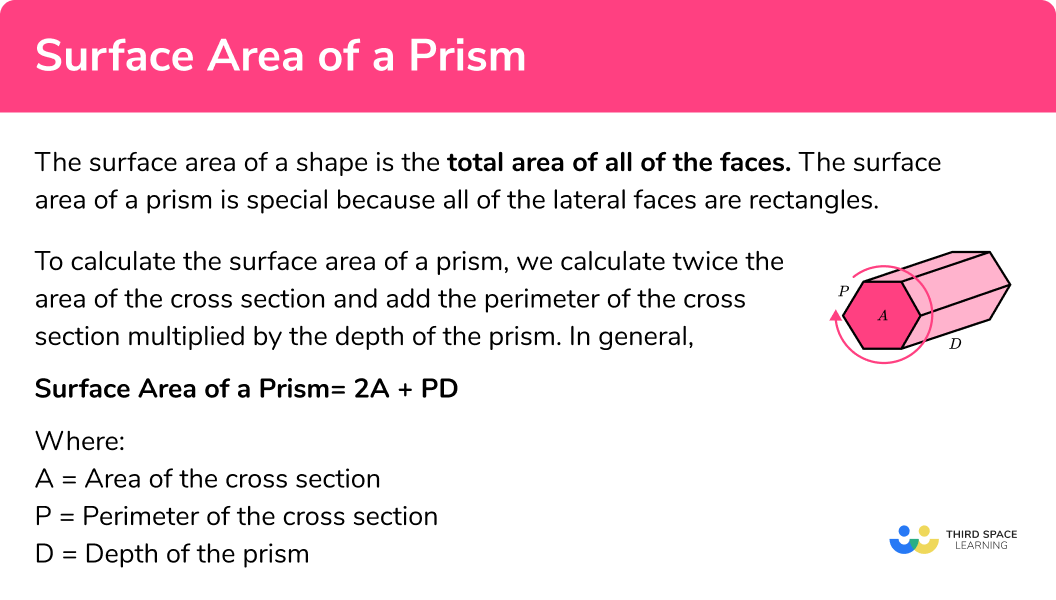## How to calculate the surface area of a prism

In order to work out the surface area of a prism:

1. Calculate the area of the cross section.
2. Calculate the perimeter of the cross section.
3. Substitute all known values into the formula and solve.
4. Write the answer, including the units.

### How to calculate the surface area of a prism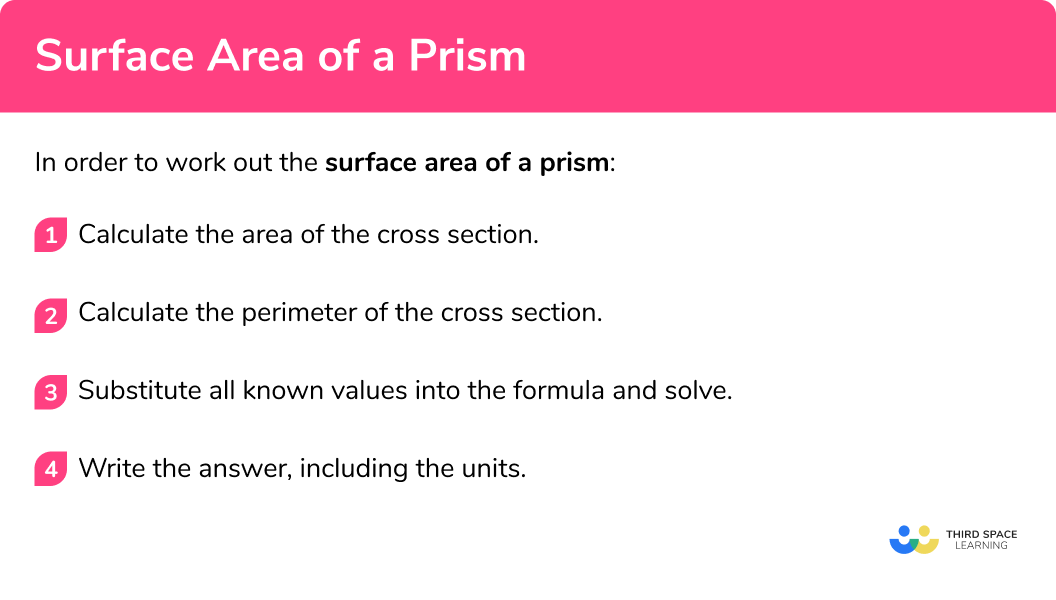## Surface area of a prism examples

### Example 4: surface area using a formula

Calculate the surface area of the following triangular prism.

1. Calculate the area of the cross section.

The cross section is a right angle triangle.

$A=\frac{1}{2}(b\times{h})\\ =\frac{1}{2}(5\times{12})\\ =30\text{cm}^{2}$

2Calculate the perimeter of the cross section.

The perimeter is the sum of the edges of the cross section:

P=5+12+13=30\text{cm}

3Substitute all known values into the formula and solve.

Using the formula Surface Area of a Prism = 2A+PD , when A=30, P=30, and D=10 , we have

$\text{SA}=2\times{30}+(30\times{10})\\ =60+300\\ =360.$

4Write the answer, including the units.

The surface area of the triangular prism is 360cm^2 .

### Example 5: surface area of a cylinder

A cylinder has a height of 5m and a radius of 2m . Calculate the surface area of the cylinder. Write your answer in terms of \pi .

1. Calculate the area of the cross section.

The cross section is a circle.

$A=\pi\times{r}^{2}\\ =\pi\times{2}^{2}\\ =4\pi\text{ m}^{2}$

2Calculate the perimeter of the cross section.

The perimeter of a circle is the circumference:

P=\pi\times{2r}=\pi\times{2}\times{2}=4\pi\text{ m}

3Substitute all known values into the formula and solve.

Using the formula Surface Area of a Prism = 2A+PD , when A=4, P=4, and D=5 , we have

$\text{SA}=2\times{4\pi}+(4\pi\times{5})\\ =8\pi+20\pi\\ =28\pi.$

4Write the answer, including the units.

The surface area of the triangular prism is 28\pi\text{ m}^{2}

### Example 6: surface area of a compound prism

Calculate the surface area of the compound prism below.

1. Calculate the area of the cross section.

The cross section is a kite.

$A=\frac{1}{2}(w\times{h})\\ =\frac{1}{2}(16\times{21})\\ =168\text{cm}^{2}$

2Calculate the perimeter of the cross section.

The perimeter is the sum of the edges of the cross section:

P=10+17+17+10=54\text{cm}

3Substitute all known values into the formula and solve.

Using the formula Surface Area of a Prism = 2A+PD , when A=168, P=54, and D=4, we have

$\text{SA}=2\times{168}+(54\times{4})\\ =336+216\\ =552.$

4Write the answer, including the units.

The surface area of the compound prism is 552cm^2 .

### Common misconceptions

• Calculating the volume instead of the surface area of the prism

Volume and surface area are different things – volume tells us the space within the shape whereas surface area is the total area of the faces. To find surface area, work out the area of each face and add them together.

• All of the rectangles have the same area

Usually all of the rectangles have different areas (unless the shape of the cross section is a regular polygon)

• Missing faces

This usually occurs on a compound prism where it can be difficult to visualise all of the face

• Missing/incorrect units

You should always include units in your answer. Remember, volume is measured in units cubed (e.g. mm^3, cm^3, m^3 etc) and surface area is measured in units squared (e.g. mm^2, cm^2, m^2 etc)

• Calculating with different units

You need to make sure all measurements are in the same units before calculating volume or surface area. (E.g. you can’t have some in cm and some in m )

• Using the wrong formula

Be careful to apply the correct prism related formula to the correct question type.

### Practice prism shape questions

1. Work out the volume of the prism160cm^390cm^372cm^3144cm^3\text{Area of trapezium }=\frac{1}{2}(3+5)\times{4}\\ =16

\text{Volume of prism }=16\times{9}\\ =144\mathrm{cm}^{3}

2. Calculate the volume of the L-shaped prism.630cm^3990cm^3526cm^317820cm^3Area of cross section:
6 \times 5=30\\ 3\times 11=33\\ 30+33=63

\text{Volume of prism }=63 \times 10\\ =630\mathrm{cm}^{3}

3. The volume of this regular octagonal prism is 360m^3 . The area of the cross section is 45m^2 . Work out the length, L, of the prism.16200m8m0.178m6mWe know the area of the octagon is 45m^2 and the volume of the prism is 360m^3 .

\text{Volume of prism } = \text{Area of cross-section } \times \text{Depth}\\ 360=45 \times{D}\\ D=8

The depth of the prism is 8m .

4. Work out the surface area of this parallelogram prism1056m^2800m^2960m^2776m^2Work out the area of each face:

\text{Total surface area }=48+48+240+240+100+100=776\mathrm{m}^{2}

5. Work out the surface area of the prism. Write your answer in cm^2 .252000cm^228200cm^24440cm^26600cm^21.2m=120cm

\text{Total surface area }=2100+2100+8400+8400+3600+3600=28200\mathrm{cm}^{2}

6. Work out the surface area of the prism484mm^2398mm^2374mm^2286mm^2Area of cross section:
Area A: \frac{1}{2} \times 8 \times 3=12
Area B: 4\times 8=32
Total area = 12+32=44

Work out the area of each face:

\text{Total surface area }=44+44+88+44+55+55+44=374\mathrm{mm}^{2}

### Prism shape GCSE questions

1. Work out the surface area of the prism(3 marks)

\frac{1}{2}(7+17) \times 12=144
For calculating the area of the cross section (trapezium)

(1)

272, 208, 208 and 112
For calculating the area of each lateral face (rectangles)

(1)

144+144+272+208+208+112=1088\mathrm{cm}^{2}
For calculating the total surface area of the prism

(1)

2. (a) The volume of this prism is 132m^3 . Work out the height of the prism.(b) Hence calculate the surface area of the prism.

(a)
5h
For calculating the area of the cross section

(1)

5h\times 12=60h
For calculating the volume of the prism

(1)

60h=132
For forming an equation to calculate the height of the prism

(1)

h=132\div{60}=2.2\text{m}

(1)

(b)
5 \times 2.2=11
For calculating the area of the cross section

(1)

60(T), 60(B), 36(L), 36(R)
For calculating the area of the rectangular faces

(1)

\text{Total surface area: }11+11+60+60+36+36=214\mathrm{m}^{2}
For calculating the total surface area of the prism

(1)

3. Richard would like to fill the container below with apple juice.Can Richard pour 5 litres of apple juice into the container without it overflowing?

(4 marks)

\frac{1}{2} (12+16) \times 12=168
For calculating the area of the cross section (trapezium)

(1)

168 \times 25 = 4200\mathrm{cm}^{3}
For calculating the volume of the container

(1)

4200\mathrm{cm}^{3}=4.2\text{ litres}
For converting the volume into litres

(1)

No, it will not fit
For correct conclusion

(1)

## Learning checklist

You have now learned how to:

• Derive and apply formulae to calculate and solve problems involving prisms
• Use the properties of faces, surfaces, edges and vertices of prisms to solve problems in 3-D
• Calculate surface areas and volumes of composite solids

## Still stuck?

Prepare your KS4 students for maths GCSEs success with Third Space Learning. Weekly online one to one GCSE maths revision lessons delivered by expert maths tutors.

Find out more about our GCSE maths tuition programme.